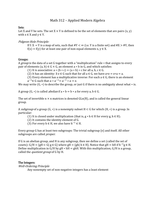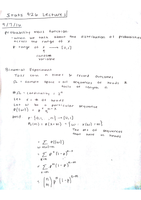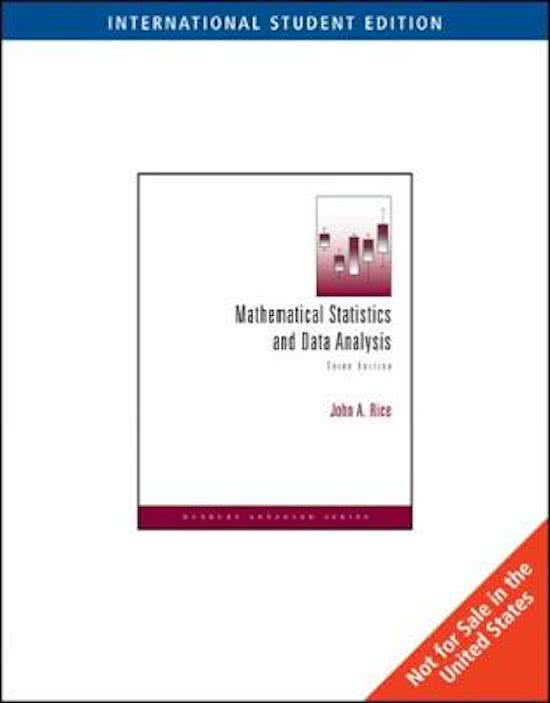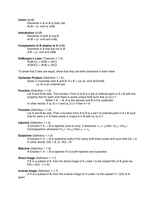# Bethanyhaefner

On this page, you find all documents, bundles, and flashcards offered by seller bethanyhaefner.

• Followers
• Following

### 3 items#### Math 312 (Modern Algebra) University of Michigan

(0)
\$6.49
0x  sold

Key concepts, definitions and theorems covered in an introductory course in modern algebra. Math 312 at the University of Michigan.

i x
• Class notes
•  • 12 pages •
• by bethanyhaefner •
Quick View
i x
•#### Stats 426 (Theoretical Statistics) University of Michigan

(0)
\$10.49
0x  sold

Discrete and continuous random variables, binomial distribution, simple random sampling with and without replacement, cumulative distribution functions, uniform distribution, inverse distribution function, exponential distribution, change of variables, bivariate distribution, conditional distribution, marginal and joint distributions, expectation, variance, standard deviation, covariance and correlation, conditional expectation, normal distribution,

i x
•  Book
• Class notes
•  • 34 pages •
• by bethanyhaefner •
Quick View
i x
•
•#### Math 451 Real Analysis / Advanced Calculus (University of Michigan)

(0)
\$7.49
0x  sold

Key definitions and theorems in analysis. University of Michigan Math 451 (Advanced Calculus).

i x
• Class notes
•  • 13 pages •
• by bethanyhaefner •